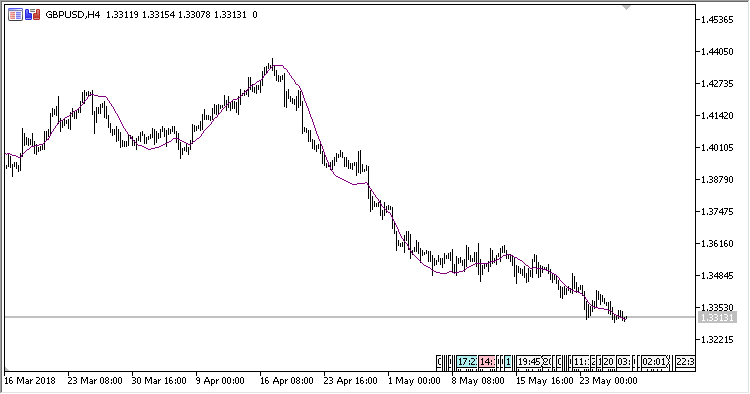# Kalman_Filter – indicator for MetaTrader 5

Indicator Kalman Filter. It allows efficiently smoothing the noise, extracting the main trend from it.

Kalman Filter is a variety of recursive filters. To assess the system status as of the current operation tact, it needs a status assessment (as the system status assessment and the error assessment of defining this status) at the preceding operation tact, and measuring at the current tact. This property distinguishes it from packet filters requiring to know at the current operation tact the history of measurements and/or assessments.

More about Kalman Filter.

The indicator has three input parameters:

• K – Kalman factor;
• Sharpness – factor for calculating the error minimization;
• Applied price – price used for calculations.
Related Posts

Calculations:

`Kalman[i] = Error + Velocity[i]`

where:

```Error = Kalman[i-1] + Distance * ShK
Velocity[i] = Velocity[i-1] + Distance * K / 100
Distance = Price[i] - Kalman[i-1]
ShK = sqrt(Sharpness * K / 100)
```